# Python: Fun with Central Tendency

Now numpy provides easy functions for finding central tendency – you can find them in my Numpy Part II lesson: Python: Numpy Part II.

But we have learned enough about Python so far, that maybe it would be more fun to build our own functions. In this lesson we are going to build our own statistics library with mean, median, mode, and quantile

## Our Data## Mean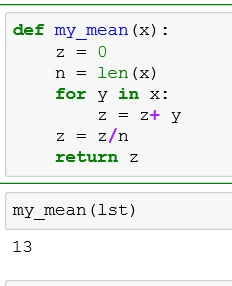or even easier: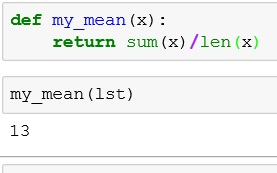## Median

Remember with median – if your data contains an odd number of elements (n%2==1), you just take the middle value. However, if your data contains and even number of elements (n%2==0) then you add the two middle numbers and divide by 2.

We handle that through the use of an if statement in our function.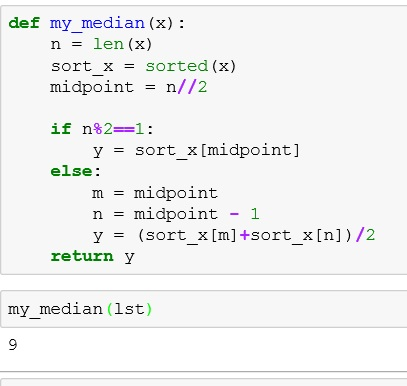## Mode

For mode, we want to find the most common element in the list. For this task, I will import Counter from collections.

d.most_common() returns each unique element and the number of times it appears

d.most_common(1) returns the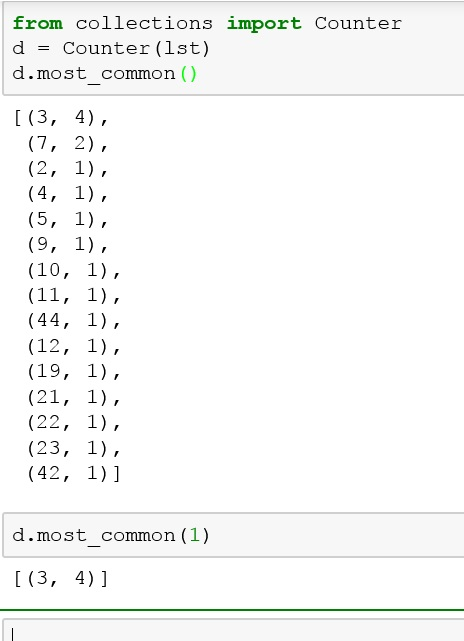or in function form: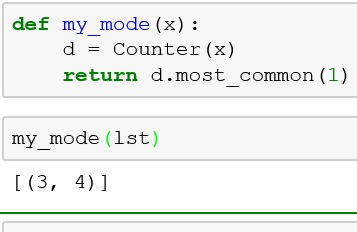In my numpy part II lesson I use a more elegant solution, but I want you to see that there is always more than one way to do something in Python.

## Quantile

Quantiles are cut points in set of data. They can represent the bottom ten percent of the data or the top 75% or any % from 0 to 100.

In my solution, I am adding a slicing argument to the sorted() function. Below shows all elements up to (but not including) index 4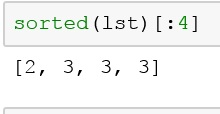quantile function:If you enjoyed this lesson, click LIKE below, or even better, leave me a COMMENT.